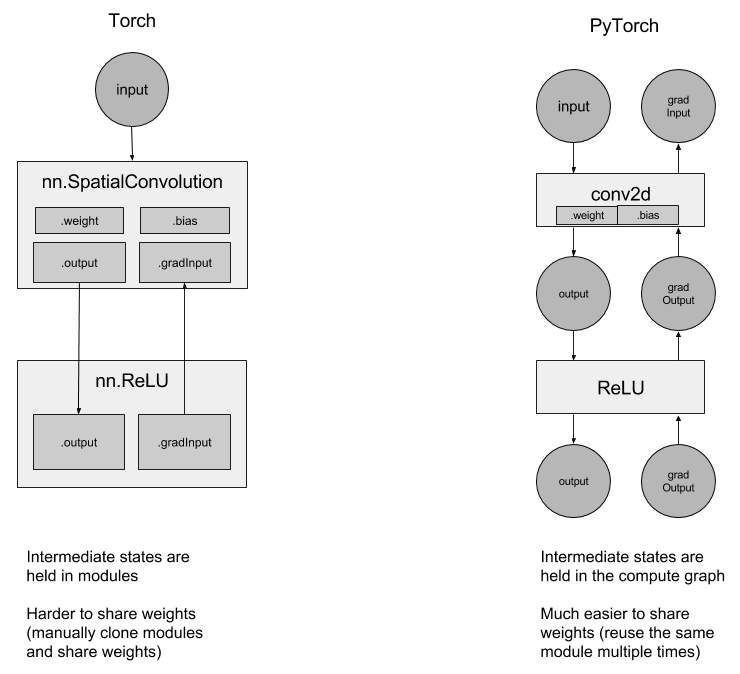# nn package¶

We’ve redesigned the nn package, so that it’s fully integrated with autograd. Let’s review the changes.

You no longer have to use Containers like ConcatTable, or modules like CAddTable, or use and debug with nngraph. We will seamlessly use autograd to define our neural networks. For example,

• output = nn.CAddTable():forward({input1, input2}) simply becomes output = input1 + input2
• output = nn.MulConstant(0.5):forward(input) simply becomes output = input * 0.5

State is no longer held in the module, but in the network graph:

Using recurrent networks should be simpler because of this reason. If you want to create a recurrent network, simply use the same Linear layer multiple times, without having to think about sharing weights.torch-nn-vs-pytorch-nn

Simplified debugging:

Debugging is intuitive using Python’s pdb debugger, and the debugger and stack traces stop at exactly where an error occurred. What you see is what you get.

## Example 1: ConvNet¶

Let’s see how to create a small ConvNet.

All of your networks are derived from the base class nn.Module:

• In the constructor, you declare all the layers you want to use.
• In the forward function, you define how your model is going to be run, from input to output
import torch
import torch.nn as nn
import torch.nn.functional as F

class MNISTConvNet(nn.Module):

def __init__(self):
# this is the place where you instantiate all your modules
# you can later access them using the same names you've given them in
# here
super(MNISTConvNet, self).__init__()
self.conv1 = nn.Conv2d(1, 10, 5)
self.pool1 = nn.MaxPool2d(2, 2)
self.conv2 = nn.Conv2d(10, 20, 5)
self.pool2 = nn.MaxPool2d(2, 2)
self.fc1 = nn.Linear(320, 50)
self.fc2 = nn.Linear(50, 10)

# it's the forward function that defines the network structure
# we're accepting only a single input in here, but if you want,
# feel free to use more
def forward(self, input):
x = self.pool1(F.relu(self.conv1(input)))
x = self.pool2(F.relu(self.conv2(x)))

# in your model definition you can go full crazy and use arbitrary
# python code to define your model structure
# all these are perfectly legal, and will be handled correctly
# if x.gt(0) > x.numel() / 2:
#      ...
#
# you can even do a loop and reuse the same module inside it
# modules no longer hold ephemeral state, so you can use them
# multiple times during your forward pass
# while x.norm(2) < 10:
#    x = self.conv1(x)

x = x.view(x.size(0), -1)
x = F.relu(self.fc1(x))
x = F.relu(self.fc2(x))
return x


Let’s use the defined ConvNet now. You create an instance of the class first.

net = MNISTConvNet()
print(net)


Note

torch.nn only supports mini-batches The entire torch.nn package only supports inputs that are a mini-batch of samples, and not a single sample.

For example, nn.Conv2d will take in a 4D Tensor of nSamples x nChannels x Height x Width.

If you have a single sample, just use input.unsqueeze(0) to add a fake batch dimension.

Create a mini-batch containing a single sample of random data and send the sample through the ConvNet.

input = Variable(torch.randn(1, 1, 28, 28))
out = net(input)
print(out.size())


Define a dummy target label and compute error using a loss function.

target = Variable(torch.LongTensor())
loss_fn = nn.CrossEntropyLoss()  # LogSoftmax + ClassNLL Loss
err = loss_fn(out, target)
err.backward()

print(err)


The output of the ConvNet out is a Variable. We compute the loss using that, and that results in err which is also a Variable. Calling .backward on err hence will propagate gradients all the way through the ConvNet to it’s weights

Let’s access individual layer weights and gradients:

print(net.conv1.weight.grad.size())

print(net.conv1.weight.data.norm())  # norm of the weight


## Forward and Backward Function Hooks¶

We’ve inspected the weights and the gradients. But how about inspecting / modifying the output and grad_output of a layer?

We introduce hooks for this purpose.

You can register a function on a Module or a Variable. The hook can be a forward hook or a backward hook. The forward hook will be executed when a forward call is executed. The backward hook will be executed in the backward phase. Let’s look at an example.

We register a forward hook on conv2 and print some information

def printnorm(self, input, output):
# input is a tuple of packed inputs
# output is a Variable. output.data is the Tensor we are interested
print('Inside ' + self.__class__.__name__ + ' forward')
print('')
print('input: ', type(input))
print('input: ', type(input))
print('output: ', type(output))
print('')
print('input size:', input.size())
print('output size:', output.data.size())
print('output norm:', output.data.norm())

net.conv2.register_forward_hook(printnorm)

out = net(input)


We register a backward hook on conv2 and print some information

def printgradnorm(self, grad_input, grad_output):
print('Inside ' + self.__class__.__name__ + ' backward')
print('Inside class:' + self.__class__.__name__)
print('')
print('')

out = net(input)
err = loss_fn(out, target)
err.backward()


A full and working MNIST example is located here https://github.com/pytorch/examples/tree/master/mnist

## Example 2: Recurrent Net¶

Next, let’s look at building recurrent nets with PyTorch.

Since the state of the network is held in the graph and not in the layers, you can simply create an nn.Linear and reuse it over and over again for the recurrence.

class RNN(nn.Module):

# you can also accept arguments in your model constructor
def __init__(self, data_size, hidden_size, output_size):
super(RNN, self).__init__()

self.hidden_size = hidden_size
input_size = data_size + hidden_size

self.i2h = nn.Linear(input_size, hidden_size)
self.h2o = nn.Linear(hidden_size, output_size)

def forward(self, data, last_hidden):
input = torch.cat((data, last_hidden), 1)
hidden = self.i2h(input)
output = self.h2o(hidden)
return hidden, output

rnn = RNN(50, 20, 10)


A more complete Language Modeling example using LSTMs and Penn Tree-bank is located here

PyTorch by default has seamless CuDNN integration for ConvNets and Recurrent Nets

loss_fn = nn.MSELoss()

batch_size = 10
TIMESTEPS = 5

# Create some fake data
batch = Variable(torch.randn(batch_size, 50))
hidden = Variable(torch.zeros(batch_size, 20))
target = Variable(torch.zeros(batch_size, 10))

loss = 0
for t in range(TIMESTEPS):
# yes! you can reuse the same network several times,
# sum up the losses, and call backward!
hidden, output = rnn(batch, hidden)
loss += loss_fn(output, target)
loss.backward()


Total running time of the script: ( 0 minutes 0.000 seconds)

Generated by Sphinx-Gallery